# class TSpectrum: public TNamed

```
```

This class contains advanced spectra processing functions for:
• One-dimensional background estimation
• One-dimensional smoothing
• One-dimensional deconvolution
• One-dimensional peak search

Author:

Miroslav Morhac
Institute of Physics
Dubravska cesta 9, 842 28 BRATISLAVA
SLOVAKIA
email:fyzimiro@savba.sk, fax:+421 7 54772479

The original code in C has been repackaged as a C++ class by R.Brun.

The algorithms in this class have been published in the following references:

1. M.Morhac et al.: Background elimination methods for multidimensional coincidence gamma-ray spectra. Nuclear Instruments and Methods in Physics Research A 401 (1997) 113-132.
2. M.Morhac et al.: Efficient one- and two-dimensional Gold deconvolution and its application to gamma-ray spectra decomposition. Nuclear Instruments and Methods in Physics Research A 401 (1997) 385-408.
3. M.Morhac et al.: Identification of peaks in multidimensional coincidence gamma-ray spectra. Nuclear Instruments and Methods in Research Physics A 443(2000), 108-125.
These NIM papers are also available as doc or ps files from:
```
```

## Function Members (Methods)

public:
 TSpectrum() TSpectrum(Int_t maxpositions, Float_t resolution = 1) virtual ~TSpectrum() void TObject::AbstractMethod(const char* method) const virtual void TObject::AppendPad(Option_t* option = "") virtual TH1* Background(const TH1* hist, Int_t niter = 20, Option_t* option = "") const char* Background(float* spectrum, Int_t ssize, Int_t numberIterations, Int_t direction, Int_t filterOrder, bool smoothing, Int_t smoothWindow, bool compton) virtual void TObject::Browse(TBrowser* b) static TClass* Class() virtual const char* TObject::ClassName() const virtual void TNamed::Clear(Option_t* option = "") virtual TObject* TNamed::Clone(const char* newname = "") const virtual Int_t TNamed::Compare(const TObject* obj) const virtual void TNamed::Copy(TObject& named) const const char* Deconvolution(float* source, const float* response, Int_t ssize, Int_t numberIterations, Int_t numberRepetitions, Double_t boost) const char* DeconvolutionRL(float* source, const float* response, Int_t ssize, Int_t numberIterations, Int_t numberRepetitions, Double_t boost) virtual void TObject::Delete(Option_t* option = "")MENU virtual Int_t TObject::DistancetoPrimitive(Int_t px, Int_t py) virtual void TObject::Draw(Option_t* option = "") virtual void TObject::DrawClass() constMENU virtual TObject* TObject::DrawClone(Option_t* option = "") constMENU virtual void TObject::Dump() constMENU virtual void TObject::Error(const char* method, const char* msgfmt) const virtual void TObject::Execute(const char* method, const char* params, Int_t* error = 0) virtual void TObject::Execute(TMethod* method, TObjArray* params, Int_t* error = 0) virtual void TObject::ExecuteEvent(Int_t event, Int_t px, Int_t py) virtual void TObject::Fatal(const char* method, const char* msgfmt) const virtual void TNamed::FillBuffer(char*& buffer) virtual TObject* TObject::FindObject(const char* name) const virtual TObject* TObject::FindObject(const TObject* obj) const virtual Option_t* TObject::GetDrawOption() const static Long_t TObject::GetDtorOnly() TH1* GetHistogram() const virtual const char* TObject::GetIconName() const virtual const char* TNamed::GetName() const Int_t GetNPeaks() const virtual char* TObject::GetObjectInfo(Int_t px, Int_t py) const static Bool_t TObject::GetObjectStat() virtual Option_t* TObject::GetOption() const Float_t* GetPositionX() const Float_t* GetPositionY() const virtual const char* TNamed::GetTitle() const virtual UInt_t TObject::GetUniqueID() const virtual Bool_t TObject::HandleTimer(TTimer* timer) virtual ULong_t TNamed::Hash() const virtual void TObject::Info(const char* method, const char* msgfmt) const virtual Bool_t TObject::InheritsFrom(const char* classname) const virtual Bool_t TObject::InheritsFrom(const TClass* cl) const virtual void TObject::Inspect() constMENU void TObject::InvertBit(UInt_t f) virtual TClass* IsA() const virtual Bool_t TObject::IsEqual(const TObject* obj) const virtual Bool_t TObject::IsFolder() const Bool_t TObject::IsOnHeap() const virtual Bool_t TNamed::IsSortable() const Bool_t TObject::IsZombie() const virtual void TNamed::ls(Option_t* option = "") const void TObject::MayNotUse(const char* method) const virtual Bool_t TObject::Notify() void TObject::Obsolete(const char* method, const char* asOfVers, const char* removedFromVers) const static void TObject::operator delete(void* ptr) static void TObject::operator delete(void* ptr, void* vp) static void TObject::operator delete[](void* ptr) static void TObject::operator delete[](void* ptr, void* vp) void* TObject::operator new(size_t sz) void* TObject::operator new(size_t sz, void* vp) void* TObject::operator new[](size_t sz) void* TObject::operator new[](size_t sz, void* vp) virtual void TObject::Paint(Option_t* option = "") virtual void TObject::Pop() virtual void Print(Option_t* option = "") const virtual Int_t TObject::Read(const char* name) virtual void TObject::RecursiveRemove(TObject* obj) void TObject::ResetBit(UInt_t f) virtual void TObject::SaveAs(const char* filename = "", Option_t* option = "") constMENU virtual void TObject::SavePrimitive(ostream& out, Option_t* option = "") virtual Int_t Search(const TH1* hist, Double_t sigma = 2, Option_t* option = "", Double_t threshold = 0.05) Int_t Search1HighRes(float* source, float* destVector, Int_t ssize, float sigma, Double_t threshold, bool backgroundRemove, Int_t deconIterations, bool markov, Int_t averWindow) Int_t SearchHighRes(float* source, float* destVector, Int_t ssize, float sigma, Double_t threshold, bool backgroundRemove, Int_t deconIterations, bool markov, Int_t averWindow) static void SetAverageWindow(Int_t w = 3) void TObject::SetBit(UInt_t f) void TObject::SetBit(UInt_t f, Bool_t set) static void SetDeconIterations(Int_t n = 3) virtual void TObject::SetDrawOption(Option_t* option = "")MENU static void TObject::SetDtorOnly(void* obj) virtual void TNamed::SetName(const char* name)MENU virtual void TNamed::SetNameTitle(const char* name, const char* title) static void TObject::SetObjectStat(Bool_t stat) void SetResolution(Float_t resolution = 1) virtual void TNamed::SetTitle(const char* title = "")MENU virtual void TObject::SetUniqueID(UInt_t uid) virtual void ShowMembers(TMemberInspector&) virtual Int_t TNamed::Sizeof() const const char* SmoothMarkov(float* source, Int_t ssize, Int_t averWindow) static TH1* StaticBackground(const TH1* hist, Int_t niter = 20, Option_t* option = "") static Int_t StaticSearch(const TH1* hist, Double_t sigma = 2, Option_t* option = "goff", Double_t threshold = 0.05) virtual void Streamer(TBuffer&) void StreamerNVirtual(TBuffer& ClassDef_StreamerNVirtual_b) virtual void TObject::SysError(const char* method, const char* msgfmt) const Bool_t TObject::TestBit(UInt_t f) const Int_t TObject::TestBits(UInt_t f) const const char* Unfolding(float* source, const float** respMatrix, Int_t ssizex, Int_t ssizey, Int_t numberIterations, Int_t numberRepetitions, Double_t boost) virtual void TObject::UseCurrentStyle() virtual void TObject::Warning(const char* method, const char* msgfmt) const virtual Int_t TObject::Write(const char* name = 0, Int_t option = 0, Int_t bufsize = 0) virtual Int_t TObject::Write(const char* name = 0, Int_t option = 0, Int_t bufsize = 0) const
protected:
 virtual void TObject::DoError(int level, const char* location, const char* fmt, va_list va) const void TObject::MakeZombie()
private:
 TSpectrum(const TSpectrum&) TSpectrum& operator=(const TSpectrum&)

## Data Members

public:
 enum { kBackOrder2 kBackOrder4 kBackOrder6 kBackOrder8 kBackIncreasingWindow kBackDecreasingWindow kBackSmoothing3 kBackSmoothing5 kBackSmoothing7 kBackSmoothing9 kBackSmoothing11 kBackSmoothing13 kBackSmoothing15 }; enum TObject::EStatusBits { kCanDelete kMustCleanup kObjInCanvas kIsReferenced kHasUUID kCannotPick kNoContextMenu kInvalidObject }; enum TObject::[unnamed] { kIsOnHeap kNotDeleted kZombie kBitMask kSingleKey kOverwrite kWriteDelete };
protected:
 TH1* fHistogram resulting histogram Int_t fMaxPeaks Maximum number of peaks to be found Int_t fNPeaks number of peaks found TString TNamed::fName object identifier Float_t* fPosition [fNPeaks] array of current peak positions Float_t* fPositionX [fNPeaks] X position of peaks Float_t* fPositionY [fNPeaks] Y position of peaks Float_t fResolution resolution of the neighboring peaks TString TNamed::fTitle object title static Int_t fgAverageWindow Average window of searched peaks static Int_t fgIterations Maximum number of decon iterations (default=3)

## Class Charts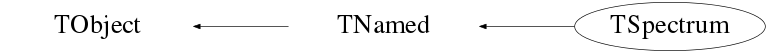## Function documentation

Constructor.

```
```
TSpectrum(Int_t maxpositions, Float_t resolution = 1)

• maxpositions: maximum number of peaks
• resolution: determines resolution of the neighboring peaks default value is 1 correspond to 3 sigma distance between peaks. Higher values allow higher resolution (smaller distance between peaks. May be set later through SetResolution.
```
```

Destructor.

```
```
void SetAverageWindow(Int_t w = 3)
Static function: Set average window of searched peaks
(see TSpectrum::SearchHighRes).

```
```
void SetDeconIterations(Int_t n = 3)
Static function: Set max number of decon iterations in deconvolution
operation (see TSpectrum::SearchHighRes).

```
```
TH1 * Background(const TH1* hist, Int_t niter = 20, Option_t* option = "")
One-dimensional background estimation function.

This function calculates the background spectrum in the input histogram h. The background is returned as a histogram.

Function parameters:

• h: input 1-d histogram
• numberIterations, (default value = 20) Increasing numberIterations make the result smoother and lower.
• option: may contain one of the following options:
• to set the direction parameter "BackIncreasingWindow". By default the direction is BackDecreasingWindow
• filterOrder-order of clipping filter, (default "BackOrder2") -possible values= "BackOrder4" "BackOrder6" "BackOrder8"
• "nosmoothing"- if selected, the background is not smoothed By default the background is smoothed.
• smoothWindow-width of smoothing window, (default is "BackSmoothing3") -possible values= "BackSmoothing5" "BackSmoothing7" "BackSmoothing9" "BackSmoothing11" "BackSmoothing13" "BackSmoothing15"
• "Compton" if selected the estimation of Compton edge will be included.
• "same" : if this option is specified, the resulting background histogram is superimposed on the picture in the current pad.
NOTE that the background is only evaluated in the current range of h. ie, if h has a bin range (set via h->GetXaxis()->SetRange(binmin,binmax), the returned histogram will be created with the same number of bins as the input histogram h, but only bins from binmin to binmax will be filled with the estimated background.
```
```
void Print(Option_t* option = "") const
Print the array of positions.

```
```
Int_t Search(const TH1* hist, Double_t sigma = 2, Option_t* option = "", Double_t threshold = 0.05)
One-dimensional peak search function

This function searches for peaks in source spectrum in hin The number of found peaks and their positions are written into the members fNpeaks and fPositionX. The search is performed in the current histogram range.

Function parameters:

• hin: pointer to the histogram of source spectrum
• sigma: sigma of searched peaks, for details we refer to manual
• threshold: (default=0.05) peaks with amplitude less than threshold*highest_peak are discarded. 0 By default, the background is removed before deconvolution. Specify the option "nobackground" to not remove the background.

By default the "Markov" chain algorithm is used. Specify the option "noMarkov" to disable this algorithm Note that by default the source spectrum is replaced by a new spectrum

By default a polymarker object is created and added to the list of functions of the histogram. The histogram is drawn with the specified option and the polymarker object drawn on top of the histogram. The polymarker coordinates correspond to the npeaks peaks found in the histogram.

A pointer to the polymarker object can be retrieved later via:

```    TList *functions = hin->GetListOfFunctions();
TPolyMarker *pm = (TPolyMarker*)functions->FindObject("TPolyMarker");
```
Specify the option "goff" to disable the storage and drawing of the polymarker.

To disable the final drawing of the histogram with the search results (in case you want to draw it yourself) specify "nodraw" in the options parameter.

```
```
void SetResolution(Float_t resolution = 1)
resolution: determines resolution of the neighboring peaks
default value is 1 correspond to 3 sigma distance
between peaks. Higher values allow higher resolution
(smaller distance between peaks.
May be set later through SetResolution.

```
```
const char * Background(float* spectrum, Int_t ssize, Int_t numberIterations, Int_t direction, Int_t filterOrder, bool smoothing, Int_t smoothWindow, bool compton)
This function calculates background spectrum from source spectrum.
The result is placed in the vector pointed by spe1945ctrum pointer.
The goal is to separate the useful information (peaks) from useless
information (background).
• method is based on Sensitive Nonlinear Iterative Peak (SNIP) clipping algorithm.
• new value in the channel "i" is calculatedwhere p = 1, 2, ..., numberIterations. In fact it represents second order difference filter (-1,2,-1). One can also change the direction of the change of the clipping window, the order of the clipping filter, to include smoothing, to set width of smoothing window and to include the estimation of Compton edges. On successful completion it returns 0. On error it returns pointer to the string describing error.

#### Parameters:

• spectrum: pointer to the vector of source spectrum
• ssize: length of the spectrum vector
• numberIterations: maximal width of clipping window,
• direction: direction of change of clipping window. Possible values: kBackIncreasingWindow, kBackDecreasingWindow
• filterOrder: order of clipping filter. Possible values: kBackOrder2, kBackOrder4, kBackOrder6, kBackOrder8
• smoothing: logical variable whether the smoothing operation in the estimation of background will be included. Possible values: kFALSE, kTRUE
• smoothWindow: width of smoothing window. Possible values: kBackSmoothing3, kBackSmoothing5, kBackSmoothing7, kBackSmoothing9, kBackSmoothing11, kBackSmoothing13, kBackSmoothing15.
• compton: logical variable whether the estimation of Compton edge will be included. Possible values: kFALSE, kTRUE.

#### References:

1. C. G Ryan et al.: SNIP, a statistics-sensitive background treatment for the quantitative analysis of PIXE spectra in geoscience applications. NIM, B34 (1988), 396-402.
2. M. Morháč, J. Kliman, V. Matouek, M. Veselský, I. Turzo: Background elimination methods for multidimensional gamma-ray spectra. NIM, A401 (1997) 113-132.
3. D. D. Burgess, R. J. Tervo: Background estimation for gamma-ray spectroscopy. NIM 214 (1983), 431-434.
Example 1 script Background_incr.c: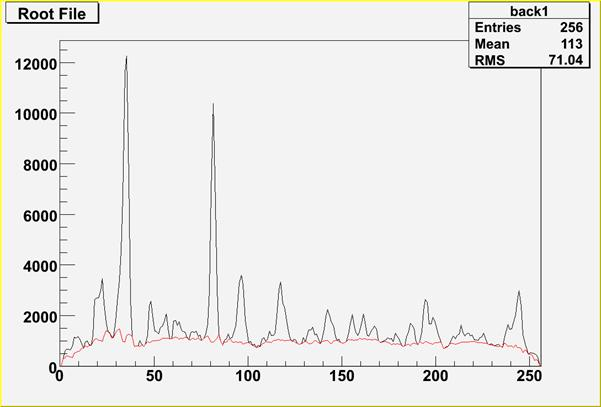Figure 1 Example of the estimation of background for number of iterations=6. Original spectrum is shown in black color, estimated background in red color.

Script:

```// Example to illustrate the background estimator (class TSpectrum).
// To execute this example, do
// root > .x Background_incr.C
#include
void Background_incr() {
Int_t i;
Double_t nbins = 256;
Double_t xmin  = 0;
Double_t xmax  = (Double_t)nbins;
Float_t * source = new float[nbins];
TH1F *back = new TH1F("back","",nbins,xmin,xmax);
TH1F *d = new TH1F("d","",nbins,xmin,xmax);
TFile *f = new TFile("spectra\\TSpectrum.root");
back=(TH1F*) f->Get("back1;1");
TCanvas *Background = gROOT->GetListOfCanvases()->FindObject("Background");
if (!Background) Background =
new TCanvas("Background",
"Estimation of background with increasing window",
10,10,1000,700);
back->Draw("L");
TSpectrum *s = new TSpectrum();
for (i = 0; i < nbins; i++) source[i]=back->GetBinContent(i + 1);
s->Background(source,nbins,6,kBackIncreasingWindow,kBackOrder2,kFALSE,
kBackSmoothing3,kFALSE);
for (i = 0; i < nbins; i++) d->SetBinContent(i + 1,source[i]);
d->SetLineColor(kRed);
d->Draw("SAME L");
}
```
Example 2 script Background_decr.c:

In Figure 1. one can notice that at the edges of the peaks the estimated background goes under the peaks. An alternative approach is to decrease the clipping window from a given value numberIterations to the value of one, which is presented in this example.Figure 2 Example of the estimation of background for numberIterations=6 using decreasing clipping window algorithm. Original spectrum is shown in black color, estimated background in red color.

Script:

```// Example to illustrate the background estimator (class TSpectrum).
// To execute this example, do
// root > .x Background_decr.C
#include
void Background_decr() {
Int_t i;
Double_t nbins = 256;
Double_t xmin  = 0;
Double_t xmax  = (Double_t)nbins;
Float_t * source = new float[nbins];
TH1F *back = new TH1F("back","",nbins,xmin,xmax);
TH1F *d = new TH1F("d","",nbins,xmin,xmax);
TFile *f = new TFile("spectra\\TSpectrum.root");
back=(TH1F*) f->Get("back1;1");
TCanvas *Background = gROOT->GetListOfCanvases()->FindObject("Background");
if (!Background) Background =
new TCanvas("Background","Estimation of background with decreasing window",
10,10,1000,700);
back->Draw("L");
TSpectrum *s = new TSpectrum();
for (i = 0; i < nbins; i++) source[i]=back->GetBinContent(i + 1);
s->Background(source,nbins,6,kBackDecreasingWindow,kBackOrder2,kFALSE,
kBackSmoothing3,kFALSE);
for (i = 0; i < nbins; i++) d->SetBinContent(i + 1,source[i]);
d->SetLineColor(kRed);
d->Draw("SAME L");
}
```
Example 3 script Background_width.c:

The question is how to choose the width of the clipping window, i.e., numberIterations parameter. The influence of this parameter on the estimated background is illustrated in Figure 3.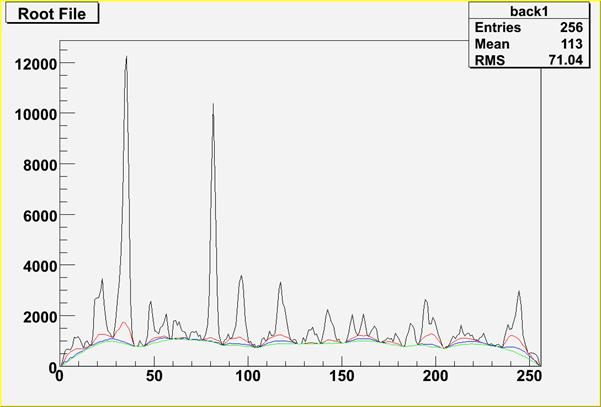Figure 3 Example of the influence of clipping window width on the estimated background for numberIterations=4 (red line), 6 (blue line) 8 (green line) using decreasing clipping window algorithm.

in general one should set this parameter so that the value 2*numberIterations+1 was greater than the widths of preserved objects (peaks).

Script:

```// Example to illustrate the influence of the clipping window width on the
// estimated background. To execute this example, do:
// root > .x Background_width.C
#include
void Background_width() {
Int_t i;
Double_t nbins = 256;
Double_t xmin  = 0;
Double_t xmax  = (Double_t)nbins;
Float_t * source = new float[nbins];
TH1F *h = new TH1F("h","",nbins,xmin,xmax);
TH1F *d1 = new TH1F("d1","",nbins,xmin,xmax);
TH1F *d2 = new TH1F("d2","",nbins,xmin,xmax);
TH1F *d3 = new TH1F("d3","",nbins,xmin,xmax);
TFile *f = new TFile("spectra\\TSpectrum.root");
h=(TH1F*) f->Get("back1;1");
TCanvas *background = gROOT->GetListOfCanvases()->FindObject("background");
if (!background) background = new TCanvas("background",
"Influence of clipping window width on the estimated background",
10,10,1000,700);
h->Draw("L");
TSpectrum *s = new TSpectrum();
for (i = 0; i < nbins; i++) source[i]=h->GetBinContent(i + 1);
s->Background(source,nbins,4,kBackDecreasingWindow,kBackOrder2,kFALSE,
kBackSmoothing3,kFALSE);
for (i = 0; i < nbins; i++) d1->SetBinContent(i + 1,source[i]);
d1->SetLineColor(kRed);
d1->Draw("SAME L");
for (i = 0; i < nbins; i++) source[i]=h->GetBinContent(i + 1);
s->Background(source,nbins,6,kBackDecreasingWindow,kBackOrder2,kFALSE,
kBackSmoothing3,kFALSE);
for (i = 0; i < nbins; i++) d2->SetBinContent(i + 1,source[i]);
d2->SetLineColor(kBlue);
d2->Draw("SAME L");
for (i = 0; i < nbins; i++) source[i]=h->GetBinContent(i + 1);
s->Background(source,nbins,8,kBackDecreasingWindow,kBackOrder2,kFALSE,
kBackSmoothing3,kFALSE);
for (i = 0; i < nbins; i++) d3->SetBinContent(i + 1,source[i]);
d3->SetLineColor(kGreen);
d3->Draw("SAME L");
}
```
Example 4 script Background_width2.c:

another example for very complex spectrum is given in Figure 4.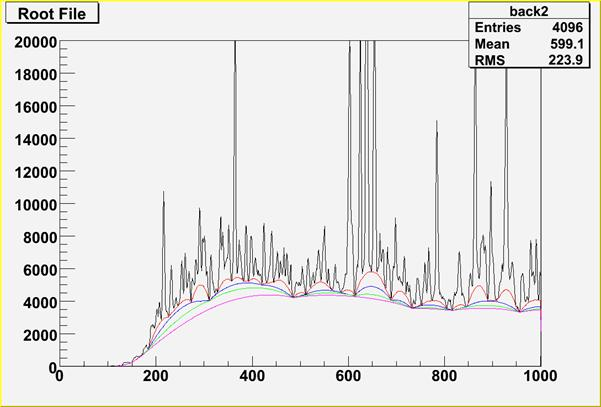Figure 4 Example of the influence of clipping window width on the estimated background for numberIterations=10 (red line), 20 (blue line), 30 (green line) and 40 (magenta line) using decreasing clipping window algorithm.

Script:

```// Example to illustrate the influence of the clipping window width on the
// estimated background. To execute this example, do:
// root > .x Background_width2.C
#include
void Background_width2() {
Int_t i;
Double_t nbins = 4096;
Double_t xmin  = 0;
Double_t xmax  = (Double_t)4096;
Float_t * source = new float[nbins];
TH1F *h = new TH1F("h","",nbins,xmin,xmax);
TH1F *d1 = new TH1F("d1","",nbins,xmin,xmax);
TH1F *d2 = new TH1F("d2","",nbins,xmin,xmax);
TH1F *d3 = new TH1F("d3","",nbins,xmin,xmax);
TH1F *d4 = new TH1F("d4","",nbins,xmin,xmax);
TFile *f = new TFile("spectra\\TSpectrum.root");
h=(TH1F*) f->Get("back2;1");
TCanvas *background = gROOT->GetListOfCanvases()->FindObject("background");
if (!background) background = new TCanvas("background",
"Influence of clipping window width on the estimated background",
10,10,1000,700);
h->SetAxisRange(0,1000);
h->SetMaximum(20000);
h->Draw("L");
TSpectrum *s = new TSpectrum();
for (i = 0; i < nbins; i++) source[i]=h->GetBinContent(i + 1);
s->Background(source,nbins,10,kBackDecreasingWindow,kBackOrder2,kFALSE,
kBackSmoothing3,kFALSE);
for (i = 0; i < nbins; i++) d1->SetBinContent(i + 1,source[i]);
d1->SetLineColor(kRed);
d1->Draw("SAME L");
for (i = 0; i < nbins; i++) source[i]=h->GetBinContent(i + 1);
s->Background(source,nbins,20,kBackDecreasingWindow,kBackOrder2,kFALSE,
kBackSmoothing3,kFALSE);
for (i = 0; i < nbins; i++) d2->SetBinContent(i + 1,source[i]);
d2->SetLineColor(kBlue);
d2->Draw("SAME L");
for (i = 0; i < nbins; i++) source[i]=h->GetBinContent(i + 1);
s->Background(source,nbins,30,kBackDecreasingWindow,kBackOrder2,kFALSE,
kBackSmoothing3,kFALSE);
for (i = 0; i < nbins; i++) d3->SetBinContent(i + 1,source[i]);
d3->SetLineColor(kGreen);
d3->Draw("SAME L");
for (i = 0; i < nbins; i++) source[i]=h->GetBinContent(i + 1);
s->Background(source,nbins,10,kBackDecreasingWindow,kBackOrder2,kFALSE,
kBackSmoothing3,kFALSE);
for (i = 0; i < nbins; i++) d4->SetBinContent(i + 1,source[i]);
d4->SetLineColor(kMagenta);
d4->Draw("SAME L");
}
```
Example 5 script Background_order.c:

Second order difference filter removes linear (quasi-linear) background and preserves symmetrical peaks. However if the shape of the background is more complex one can employ higher-order clipping filters (see example in Figure 5)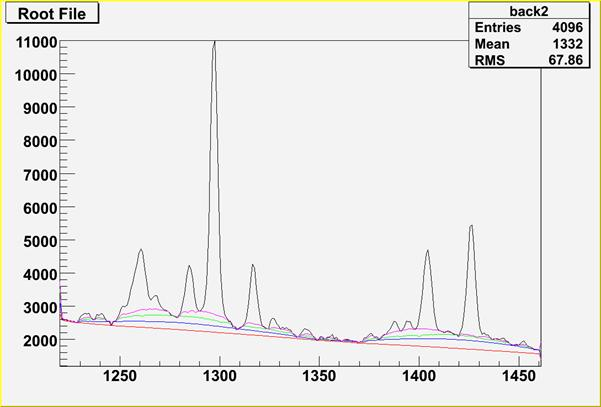Figure 5 Example of the influence of clipping filter difference order on the estimated background for fNnumberIterations=40, 2-nd order red line, 4-th order blue line, 6-th order green line and 8-th order magenta line, and using decreasing clipping window algorithm.

Script:

```// Example to illustrate the influence of the clipping filter difference order
// on the estimated background. To execute this example, do
// root > .x Background_order.C
#include
void Background_order() {
Int_t i;
Double_t nbins = 4096;
Double_t xmin  = 0;
Double_t xmax  = (Double_t)4096;
Float_t * source = new float[nbins];
TH1F *h = new TH1F("h","",nbins,xmin,xmax);
TH1F *d1 = new TH1F("d1","",nbins,xmin,xmax);
TH1F *d2 = new TH1F("d2","",nbins,xmin,xmax);
TH1F *d3 = new TH1F("d3","",nbins,xmin,xmax);
TH1F *d4 = new TH1F("d4","",nbins,xmin,xmax);
TFile *f = new TFile("spectra\\TSpectrum.root");
h=(TH1F*) f->Get("back2;1");
TCanvas *background = gROOT->GetListOfCanvases()->FindObject("background");
if (!background) background = new TCanvas("background",
"Influence of clipping filter difference order on the estimated background",
10,10,1000,700);
h->SetAxisRange(1220,1460);
h->SetMaximum(11000);
h->Draw("L");
TSpectrum *s = new TSpectrum();
for (i = 0; i < nbins; i++) source[i]=h->GetBinContent(i + 1);
s->Background(source,nbins,40,kBackDecreasingWindow,kBackOrder2,kFALSE,
kBackSmoothing3,kFALSE);
for (i = 0; i < nbins; i++) d1->SetBinContent(i + 1,source[i]);
d1->SetLineColor(kRed);
d1->Draw("SAME L");
for (i = 0; i < nbins; i++) source[i]=h->GetBinContent(i + 1);
s->Background(source,nbins,40,kBackDecreasingWindow,kBackOrder4,kFALSE,
kBackSmoothing3,kFALSE);
for (i = 0; i < nbins; i++) d2->SetBinContent(i + 1,source[i]);
d2->SetLineColor(kBlue);
d2->Draw("SAME L");
for (i = 0; i < nbins; i++) source[i]=h->GetBinContent(i + 1);
s->Background(source,nbins,40,kBackDecreasingWindow,kBackOrder6,kFALSE,
kBackSmoothing3,kFALSE);
for (i = 0; i < nbins; i++) d3->SetBinContent(i + 1,source[i]);
d3->SetLineColor(kGreen);
d3->Draw("SAME L");
for (i = 0; i < nbins; i++) source[i]=h->GetBinContent(i + 1);
s->Background(source,nbins,40,kBackDecreasingWindow,kBackOrder8,kFALSE,
kBackSmoothing3,kFALSE);
for (i = 0; i < nbins; i++) d4->SetBinContent(i + 1,source[i]);
d4->SetLineColor(kMagenta);
d4->Draw("SAME L");
}
```
Example 6 script Background_smooth.c:

The estimate of the background can be influenced by noise present in the spectrum. We proposed the algorithm of the background estimate with simultaneous smoothing. In the original algorithm without smoothing, the estimated background snatches the lower spikes in the noise. Consequently, the areas of peaks are biased by this error.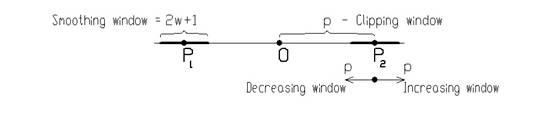Figure 7 Principle of background estimation algorithm with simultaneous smoothing.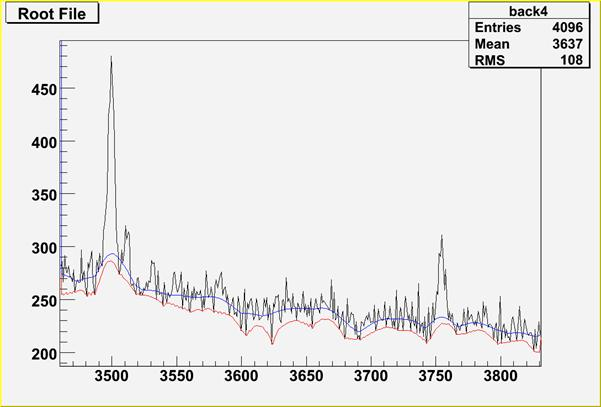Figure 8 Illustration of non-smoothing (red line) and smoothing algorithm of background estimation (blue line).

Script:

```// Example to illustrate the background estimator (class TSpectrum) including
// Compton edges. To execute this example, do:
// root > .x Background_smooth.C
#include
void Background_smooth() {
Int_t i;
Double_t nbins = 4096;
Double_t xmin  = 0;
Double_t xmax  = (Double_t)nbins;
Float_t * source = new float[nbins];
TH1F *h = new TH1F("h","",nbins,xmin,xmax);
TH1F *d1 = new TH1F("d1","",nbins,xmin,xmax);
TH1F *d2 = new TH1F("d2","",nbins,xmin,xmax);
TFile *f = new TFile("spectra\\TSpectrum.root");
h=(TH1F*) f->Get("back4;1");
TCanvas *background = gROOT->GetListOfCanvases()->FindObject("background");
if (!background) background = new TCanvas("background",
"Estimation of background with noise",10,10,1000,700);
h->SetAxisRange(3460,3830);
h->Draw("L");
TSpectrum *s = new TSpectrum();
for (i = 0; i < nbins; i++) source[i]=h->GetBinContent(i + 1);
s->Background(source,nbins,6,kBackDecreasingWindow,kBackOrder2,kFALSE,
kBackSmoothing3,kFALSE);
for (i = 0; i < nbins; i++) d1->SetBinContent(i + 1,source[i]);
d1->SetLineColor(kRed);
d1->Draw("SAME L");
for (i = 0; i < nbins; i++) source[i]=h->GetBinContent(i + 1);
s->Background(source,nbins,6,kBackDecreasingWindow,kBackOrder2,kTRUE,
kBackSmoothing3,kFALSE);
for (i = 0; i < nbins; i++) d2->SetBinContent(i + 1,source[i]);
d2->SetLineColor(kBlue);
d2->Draw("SAME L");
}
```
Example 8 script Background_compton.c:

Sometimes it is necessary to include also the Compton edges into the estimate of the background. In Figure 8 we present the example of the synthetic spectrum with Compton edges. The background was estimated using the 8-th order filter with the estimation of the Compton edges using decreasing clipping window algorithm (numberIterations=10) with smoothing (smoothingWindow=5).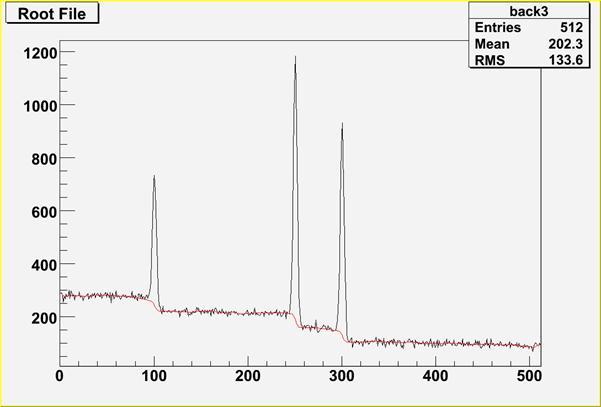Figure 8 Example of the estimate of the background with Compton edges (red line) for numberIterations=10, 8-th order difference filter, using decreasing clipping window algorithm and smoothing (smoothingWindow=5).

Script:

```// Example to illustrate the background estimator (class TSpectrum) including
// Compton edges. To execute this example, do:
// root > .x Background_compton.C
#include
void Background_compton() {
Int_t i;
Double_t nbins = 512;
Double_t xmin  = 0;
Double_t xmax  = (Double_t)nbins;
Float_t * source = new float[nbins];
TH1F *h = new TH1F("h","",nbins,xmin,xmax);
TH1F *d1 = new TH1F("d1","",nbins,xmin,xmax);
TFile *f = new TFile("spectra\\TSpectrum.root");
h=(TH1F*) f->Get("back3;1");
TCanvas *background = gROOT->GetListOfCanvases()->FindObject("background");
if (!background) background = new TCanvas("background",
"Estimation of background with Compton edges under peaks",10,10,1000,700);
h->Draw("L");
TSpectrum *s = new TSpectrum();
for (i = 0; i < nbins; i++) source[i]=h->GetBinContent(i + 1);
s->Background(source,nbins,10,kBackDecreasingWindow,kBackOrder8,kTRUE,
kBackSmoothing5,,kTRUE);
for (i = 0; i < nbins; i++) d1->SetBinContent(i + 1,source[i]);
d1->SetLineColor(kRed);
d1->Draw("SAME L");
}
```
```
```
const char* SmoothMarkov(float* source, Int_t ssize, Int_t averWindow)
One-dimensional markov spectrum smoothing function

This function calculates smoothed spectrum from source spectrum based on Markov chain method. The result is placed in the array pointed by source pointer. On successful completion it returns 0. On error it returns pointer to the string describing error.

Function parameters:

• source: pointer to the array of source spectrum
• ssize: length of source array
• averWindow: width of averaging smoothing window
The goal of this function is the suppression of the statistical fluctuations. The algorithm is based on discrete Markov chain, which has very simple invariant distribution: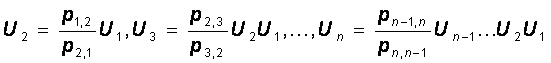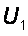being defined from the normalization condition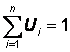. n is the length of the smoothed spectrum and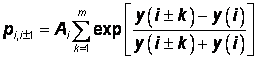Reference:

1. Z.K. Silagadze, A new algorithm for automatic photopeak searches. NIM A 376 (1996), 451.

Example 14 - script Smoothing.c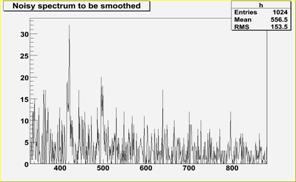Fig. 23 Original noisy spectrum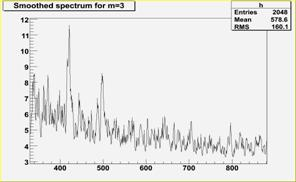Fig. 24 Smoothed spectrum m=3Fig. 25 Smoothed spectrum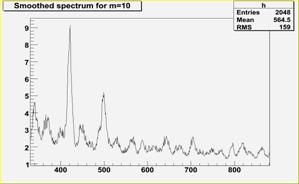Fig.26 Smoothed spectrum m=10

Script:

```   // Example to illustrate smoothing using Markov algorithm (class TSpectrum).
// To execute this example, do
// root > .x Smoothing.C
void Smoothing() {
Int_t i;
Double_t nbins = 1024;
Double_t xmin  = 0;
Double_t xmax  = (Double_t)nbins;
Float_t * source = new float[nbins];
TH1F *h = new TH1F("h","Smoothed spectrum for m=3",nbins,xmin,xmax);
TFile *f = new TFile("spectra\\TSpectrum.root");
h=(TH1F*) f->Get("smooth1;1");
for (i = 0; i < nbins; i++) source[i]=h->GetBinContent(i + 1);
TCanvas *Smooth1 = gROOT->GetListOfCanvases()->FindObject("Smooth1");
if (!Smooth1) Smooth1 = new TCanvas("Smooth1","Smooth1",10,10,1000,700);
TSpectrum *s = new TSpectrum();
s->SmoothMarkov(source,1024,3);  //3, 7, 10
for (i = 0; i < nbins; i++) h->SetBinContent(i + 1,source[i]);
h->SetAxisRange(330,880);
h->Draw("L");
}
```
```
```
const char * Deconvolution(float* source, const float* response, Int_t ssize, Int_t numberIterations, Int_t numberRepetitions, Double_t boost)
One-dimensional deconvolution function

This function calculates deconvolution from source spectrum according to response spectrum using Gold deconvolution algorithm. The result is placed in the vector pointed by source pointer. On successful completion it returns 0. On error it returns pointer to the string describing error. If desired after every numberIterations one can apply boosting operation (exponential function with exponent given by boost coefficient) and repeat it numberRepetitions times.

Function parameters:

• source: pointer to the vector of source spectrum
• response: pointer to the vector of response spectrum
• ssize: length of source and response spectra numberIterations, for details we refer to the reference given below numberRepetitions, for repeated boosted deconvolution boost, boosting coefficient
The goal of this function is the improvement of the resolution in spectra, decomposition of multiplets. The mathematical formulation of the convolution system is:where h(i) is the impulse response function, x, y are input and output vectors, respectively, N is the length of x and h vectors. In matrix form we have: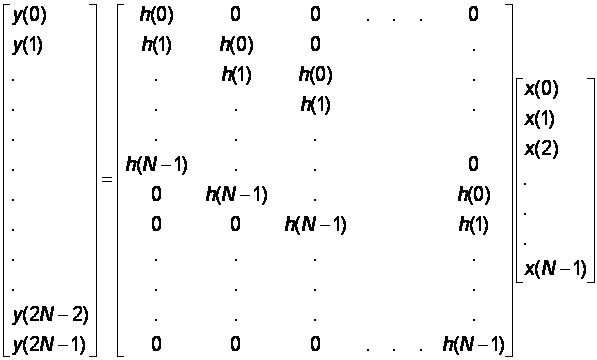Let us assume that we know the response and the output vector (spectrum) of the above given system. The deconvolution represents solution of the overdetermined system of linear equations, i.e., the calculation of the vector x. From numerical stability point of view the operation of deconvolution is extremely critical (ill-posed problem) as well as time consuming operation. The Gold deconvolution algorithm proves to work very well, other methods (Fourier, VanCittert etc) oscillate. It is suitable to process positive definite data (e.g. histograms).

Gold deconvolution algorithm:Where L is given number of iterations (numberIterations parameter).

Boosted deconvolution:

1. Set the initial solution:
```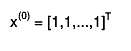$x^{(0)} = [1,1,...,1]^{T}$
```
2. Set required number of repetitions R and iterations L.
3. Set r = 1.
4. Using Gold deconvolution algorithm for k=1,2,...,L find
```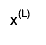$x^{(L)}$
```
5. If r = R stop calculation, else
1. Apply boosting operation, i.e., set
```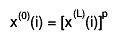$x^{(0)}(i) = [x^{(L)}(i)]^{p}$
```
i=0,1,...N-1 and p is boosting coefficient >0.
2. r = r + 1
3. continue in 4.

References:

1. Gold R., ANL-6984, Argonne National Laboratories, Argonne Ill, 1964.
2. Coote G.E., Iterative smoothing and deconvolution of one- and two-dimensional elemental distribution data, NIM B 130 (1997) 118.
3. M. Morháč, J. Kliman, V. Matouek, M. Veselský, I. Turzo: Efficient one- and two-dimensional Gold deconvolution and its application to gamma-ray spectra decomposition. NIM, A401 (1997) 385-408.
4. Morháč M., Matouek V., Kliman J., Efficient algorithm of multidimensional deconvolution and its application to nuclear data processing, Digital Signal Processing 13 (2003) 144.

Example 8 - script Deconvolution.c :

response function (usually peak) should be shifted left to the first non-zero channel (bin) (see Figure 9)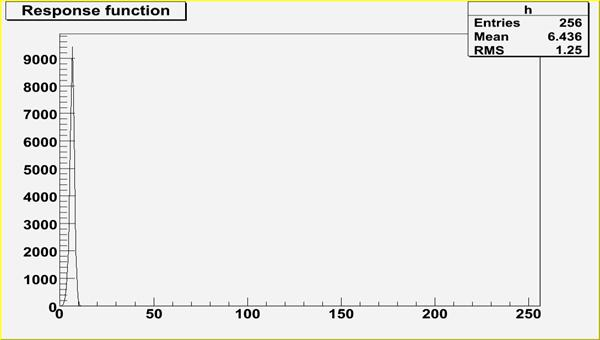Figure 9 Response spectrum.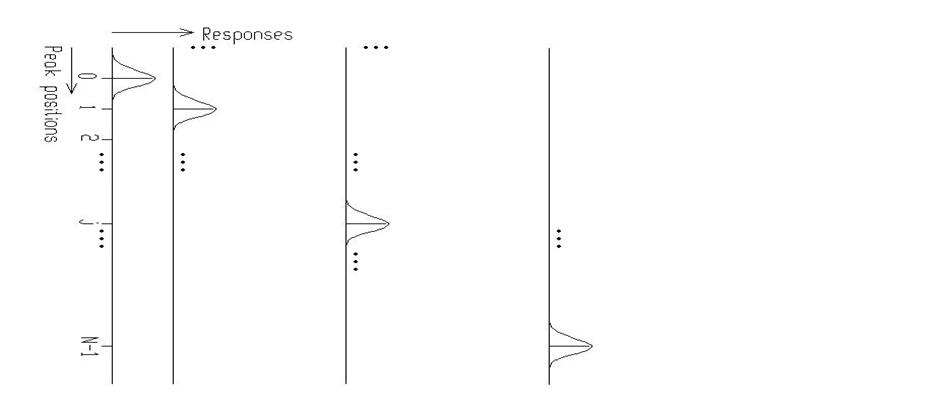Figure 10 Principle how the response matrix is composed inside of the Deconvolution function.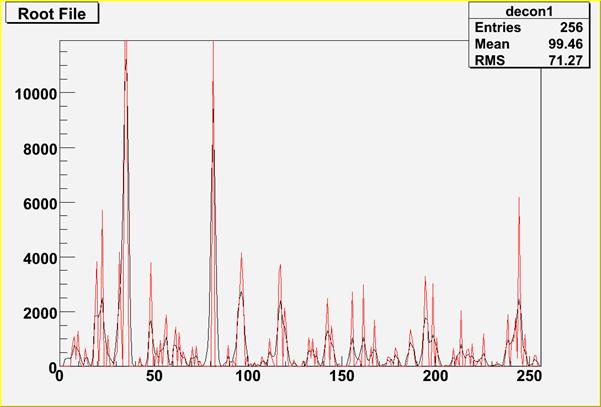Figure 11 Example of Gold deconvolution. The original source spectrum is drawn with black color, the spectrum after the deconvolution (10000 iterations) with red color.

Script:

```   // Example to illustrate deconvolution function (class TSpectrum).
// To execute this example, do
// root > .x Deconvolution.C
#include
void Deconvolution() {
Int_t i;
Double_t nbins = 256;
Double_t xmin  = 0;
Double_t xmax  = (Double_t)nbins;
Float_t * source = new float[nbins];
Float_t * response = new float[nbins];
TH1F *h = new TH1F("h","Deconvolution",nbins,xmin,xmax);
TH1F *d = new TH1F("d","",nbins,xmin,xmax);
TFile *f = new TFile("spectra\\TSpectrum.root");
h=(TH1F*) f->Get("decon1;1");
TFile *fr = new TFile("spectra\\TSpectrum.root");
d=(TH1F*) fr->Get("decon_response;1");
for (i = 0; i < nbins; i++) source[i]=h->GetBinContent(i + 1);
for (i = 0; i < nbins; i++) response[i]=d->GetBinContent(i + 1);
TCanvas *Decon1 = gROOT->GetListOfCanvases()->FindObject("Decon1");
if (!Decon1) Decon1 = new TCanvas("Decon1","Decon1",10,10,1000,700);
h->Draw("L");
TSpectrum *s = new TSpectrum();
s->Deconvolution(source,response,256,1000,1,1);
for (i = 0; i < nbins; i++) d->SetBinContent(i + 1,source[i]);
d->SetLineColor(kRed);
d->Draw("SAME L");
}
```

Examples of Gold deconvolution method:

First let us study the influence of the number of iterations on the deconvolved spectrum (Figure 12).Figure 12 Study of Gold deconvolution algorithm.The original source spectrum is drawn with black color, spectrum after 100 iterations with red color, spectrum after 1000 iterations with blue color, spectrum after 10000 iterations with green color and spectrum after 100000 iterations with magenta color.

For relatively narrow peaks in the above given example the Gold deconvolution method is able to decompose overlapping peaks practically to delta - functions. In the next example we have chosen a synthetic data (spectrum, 256 channels) consisting of 5 very closely positioned, relatively wide peaks (sigma =5), with added noise (Figure 13). Thin lines represent pure Gaussians (see Table 1); thick line is a resulting spectrum with additive noise (10% of the amplitude of small peaks).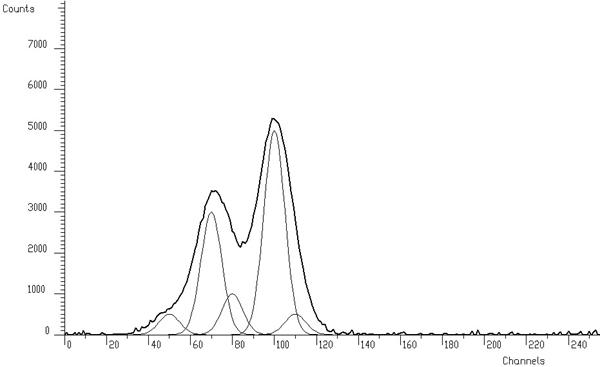Figure 13 Testing example of synthetic spectrum composed of 5 Gaussians with added noise.

 Peak # Position Height Area 1 50 500 10159 2 70 3000 60957 3 80 1000 20319 4 100 5000 101596 5 110 500 10159

Table 1 Positions, heights and areas of peaks in the spectrum shown in Figure 13.

In ideal case, we should obtain the result given in Figure 14. The areas of the Gaussian components of the spectrum are concentrated completely to delta-functions. When solving the overdetermined system of linear equations with data from Figure 13 in the sense of minimum least squares criterion without any regularization we obtain the result with large oscillations (Figure 15). From mathematical point of view, it is the optimal solution in the unconstrained space of independent variables. From physical point of view we are interested only in a meaningful solution. Therefore, we have to employ regularization techniques (e.g. Gold deconvolution) and/or to confine the space of allowed solutions to subspace of positive solutions.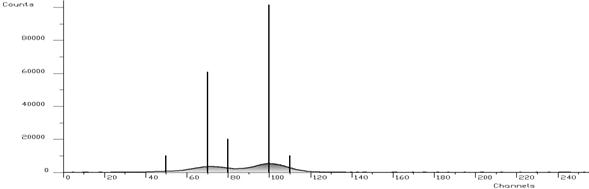Figure 14 The same spectrum like in Figure 13, outlined bars show the contents of present components (peaks).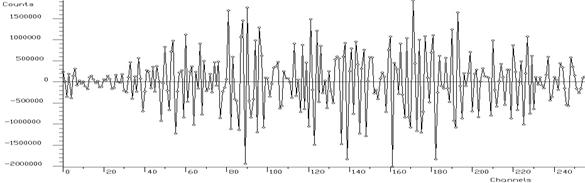Figure 15 Least squares solution of the system of linear equations without regularization.

Example 9 - script Deconvolution_wide.c

When we employ Gold deconvolution algorithm we obtain the result given in Fig. 16. One can observe that the resulting spectrum is smooth. On the other hand the method is not able to decompose completely the peaks in the spectrum.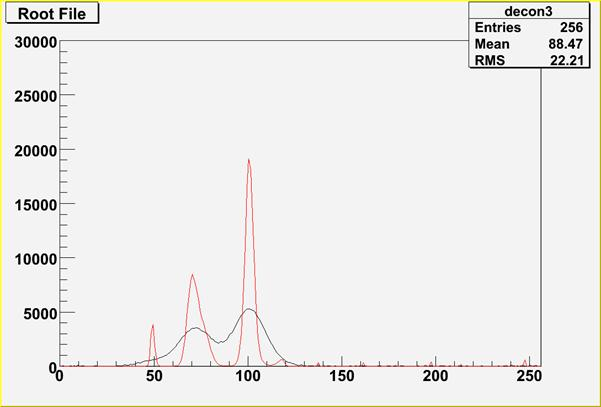Figure 16 Example of Gold deconvolution for closely positioned wide peaks. The original source spectrum is drawn with black color, the spectrum after the deconvolution (10000 iterations) with red color.

Script:

```   // Example to illustrate deconvolution function (class TSpectrum).
// To execute this example, do
// root > .x Deconvolution_wide.C
#include
void Deconvolution_wide() {
Int_t i;
Double_t nbins = 256;
Double_t xmin  = 0;
Double_t xmax  = (Double_t)nbins;
Float_t * source = new float[nbins];
Float_t * response = new float[nbins];
TH1F *h = new TH1F("h","Deconvolution",nbins,xmin,xmax);
TH1F *d = new TH1F("d","",nbins,xmin,xmax);
TFile *f = new TFile("spectra\\TSpectrum.root");
h=(TH1F*) f->Get("decon3;1");
TFile *fr = new TFile("spectra\\TSpectrum.root");
d=(TH1F*) fr->Get("decon_response_wide;1");
for (i = 0; i < nbins; i++) source[i]=h->GetBinContent(i + 1);
for (i = 0; i < nbins; i++) response[i]=d->GetBinContent(i + 1);
TCanvas *Decon1 = gROOT->GetListOfCanvases()->FindObject("Decon1");
if (!Decon1) Decon1 = new TCanvas("Decon1",
"Deconvolution of closely positioned overlapping peaks using Gold deconvolution method",10,10,1000,700);
h->SetMaximum(30000);
h->Draw("L");
TSpectrum *s = new TSpectrum();
s->Deconvolution(source,response,256,10000,1,1);
for (i = 0; i < nbins; i++) d->SetBinContent(i + 1,source[i]);
d->SetLineColor(kRed);
d->Draw("SAME L");
}
```

Example 10 - script Deconvolution_wide_boost.c :

Further let us employ boosting operation into deconvolution (Fig. 17).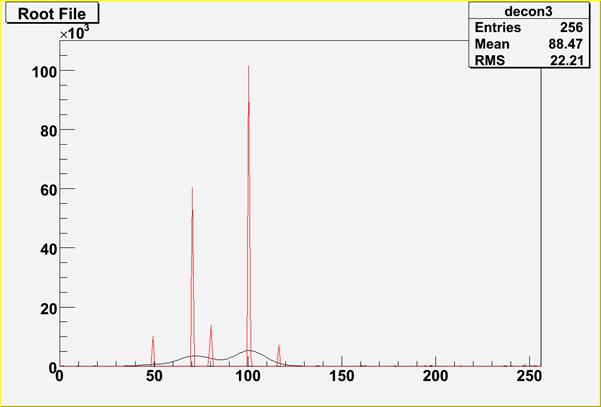Figure 17 The original source spectrum is drawn with black color, the spectrum after the deconvolution with red color. Number of iterations = 200, number of repetitions = 50 and boosting coefficient = 1.2.

 Peak # Original/Estimated (max) position Original/Estimated area 1 50/49 10159/10419 2 70/70 60957/58933 3 80/79 20319/19935 4 100/100 101596/105413 5 110/117 10159/6676

Table 2 Results of the estimation of peaks in spectrum shown in Figure 17.

One can observe that peaks are decomposed practically to delta functions. Number of peaks is correct, positions of big peaks as well as their areas are relatively well estimated. However there is a considerable error in the estimation of the position of small right hand peak.

Script:

```   // Example to illustrate deconvolution function (class TSpectrum).
// To execute this example, do
// root > .x Deconvolution_wide_boost.C
#include
void Deconvolution_wide_boost() {
Int_t i;
Double_t nbins = 256;
Double_t xmin  = 0;
Double_t xmax  = (Double_t)nbins;
Float_t * source = new float[nbins];
Float_t * response = new float[nbins];
TH1F *h = new TH1F("h","Deconvolution",nbins,xmin,xmax);
TH1F *d = new TH1F("d","",nbins,xmin,xmax);
TFile *f = new TFile("spectra\\TSpectrum.root");
h=(TH1F*) f->Get("decon3;1");
TFile *fr = new TFile("spectra\\TSpectrum.root");
d=(TH1F*) fr->Get("decon_response_wide;1");
for (i = 0; i < nbins; i++) source[i]=h->GetBinContent(i + 1);
for (i = 0; i < nbins; i++) response[i]=d->GetBinContent(i + 1);
TCanvas *Decon1 = gROOT->GetListOfCanvases()->FindObject("Decon1");
if (!Decon1) Decon1 = new TCanvas("Decon1",
"Deconvolution of closely positioned overlapping peaks using boosted Gold deconvolution method",10,10,1000,700);
h->SetMaximum(110000);
h->Draw("L");
TSpectrum *s = new TSpectrum();
s->Deconvolution(source,response,256,200,50,1.2);
for (i = 0; i < nbins; i++) d->SetBinContent(i + 1,source[i]);
d->SetLineColor(kRed);
d->Draw("SAME L");
}
```
```
```
const char * DeconvolutionRL(float* source, const float* response, Int_t ssize, Int_t numberIterations, Int_t numberRepetitions, Double_t boost)
One-dimensional deconvolution function.

This function calculates deconvolution from source spectrum according to response spectrum using Richardson-Lucy deconvolution algorithm. The result is placed in the vector pointed by source pointer. On successful completion it returns 0. On error it returns pointer to the string describing error. If desired after every numberIterations one can apply boosting operation (exponential function with exponent given by boost coefficient) and repeat it numberRepetitions times (see Gold deconvolution).

Function parameters:

• source: pointer to the vector of source spectrum
• response: pointer to the vector of response spectrum
• ssize: length of source and response spectra numberIterations, for details we refer to the reference given above numberRepetitions, for repeated boosted deconvolution boost, boosting coefficient

Richardson-Lucy deconvolution algorithm:

For discrete systems it has the form: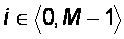for positive input data and response matrix this iterative method forces the deconvoluted spectra to be non-negative. The Richardson-Lucy iteration converges to the maximum likelihood solution for Poisson statistics in the data.

References:

1. Abreu M.C. et al., A four-dimensional deconvolution method to correct NA38 experimental data, NIM A 405 (1998) 139.
2. Lucy L.B., A.J. 79 (1974) 745.
3. Richardson W.H., J. Opt. Soc. Am. 62 (1972) 55.

Examples of Richardson-Lucy deconvolution method:

Example 11 - script DeconvolutionRL_wide.c :

When we employ Richardson-Lucy deconvolution algorithm to our data from Fig. 13 we obtain the result given in Fig. 18. One can observe improvements as compared to the result achieved by Gold deconvolution. Neverthless it is unable to decompose the multiplet.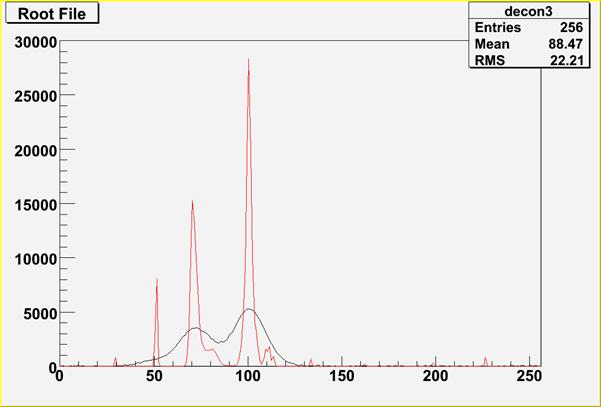Figure 18 Example of Richardson-Lucy deconvolution for closely positioned wide peaks. The original source spectrum is drawn with black color, the spectrum after the deconvolution (10000 iterations) with red color.

Script:

```   // Example to illustrate deconvolution function (class TSpectrum).
// To execute this example, do
// root > .x DeconvolutionRL_wide.C
#include
void DeconvolutionRL_wide() {
Int_t i;
Double_t nbins = 256;
Double_t xmin  = 0;
Double_t xmax  = (Double_t)nbins;
Float_t * source = new float[nbins];
Float_t * response = new float[nbins];
TH1F *h = new TH1F("h","Deconvolution",nbins,xmin,xmax);
TH1F *d = new TH1F("d","",nbins,xmin,xmax);
TFile *f = new TFile("spectra\\TSpectrum.root");
h=(TH1F*) f->Get("decon3;1");
TFile *fr = new TFile("spectra\\TSpectrum.root");
d=(TH1F*) fr->Get("decon_response_wide;1");
for (i = 0; i < nbins; i++) source[i]=h->GetBinContent(i + 1);
for (i = 0; i < nbins; i++) response[i]=d->GetBinContent(i + 1);
TCanvas *Decon1 = gROOT->GetListOfCanvases()->FindObject("Decon1");
if (!Decon1) Decon1 = new TCanvas("Decon1",
"Deconvolution of closely positioned overlapping peaks using Richardson-Lucy deconvolution method",
10,10,1000,700);
h->SetMaximum(30000);
h->Draw("L");
TSpectrum *s = new TSpectrum();
s->DeconvolutionRL(source,response,256,10000,1,1);
for (i = 0; i < nbins; i++) d->SetBinContent(i + 1,source[i]);
d->SetLineColor(kRed);
d->Draw("SAME L");
}
```

Example 12 - script DeconvolutionRL_wide_boost.c :

Further let us employ boosting operation into deconvolution (Fig. 19).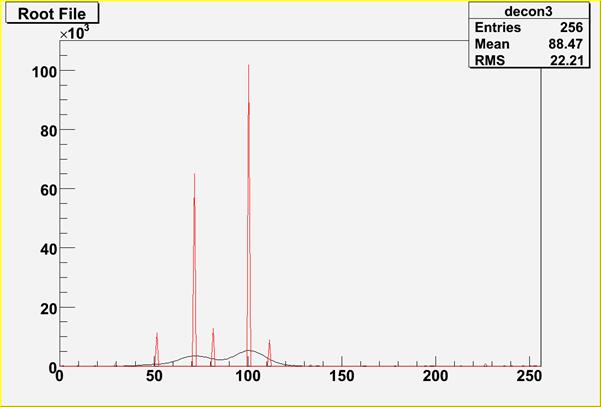Figure 19 The original source spectrum is drawn with black color, the spectrum after the deconvolution with red color. Number of iterations = 200, number of repetitions = 50 and boosting coefficient = 1.2.

 Peak # Original/Estimated (max) position Original/Estimated area 1 50/51 10159/11426 2 70/71 60957/65003 3 80/81 20319/12813 4 100/100 101596/101851 5 110/111 10159/8920

Table 3 Results of the estimation of peaks in spectrum shown in Figure 19.

One can observe improvements in the estimation of peak positions as compared to the results achieved by Gold deconvolution.

Script:

```   // Example to illustrate deconvolution function (class TSpectrum).
// To execute this example, do
// root > .x DeconvolutionRL_wide_boost.C
#include
void DeconvolutionRL_wide_boost() {
Int_t i;
Double_t nbins = 256;
Double_t xmin  = 0;
Double_t xmax  = (Double_t)nbins;
Float_t * source = new float[nbins];
Float_t * response = new float[nbins];
TH1F *h = new TH1F("h","Deconvolution",nbins,xmin,xmax);
TH1F *d = new TH1F("d","",nbins,xmin,xmax);
TFile *f = new TFile("spectra\\TSpectrum.root");
h=(TH1F*) f->Get("decon3;1");
TFile *fr = new TFile("spectra\\TSpectrum.root");
d=(TH1F*) fr->Get("decon_response_wide;1");
for (i = 0; i < nbins; i++) source[i]=h->GetBinContent(i + 1);
for (i = 0; i < nbins; i++) response[i]=d->GetBinContent(i + 1);
TCanvas *Decon1 = gROOT->GetListOfCanvases()->FindObject("Decon1");
if (!Decon1) Decon1 = new TCanvas("Decon1",
"Deconvolution of closely positioned overlapping peaks using boosted Richardson-Lucy deconvolution method",
10,10,1000,700);
h->SetMaximum(110000);
h->Draw("L");
TSpectrum *s = new TSpectrum();
s->DeconvolutionRL(source,response,256,200,50,1.2);
for (i = 0; i < nbins; i++) d->SetBinContent(i + 1,source[i]);
d->SetLineColor(kRed);
d->Draw("SAME L");
}
```
```
```
const char * Unfolding(float* source, const float** respMatrix, Int_t ssizex, Int_t ssizey, Int_t numberIterations, Int_t numberRepetitions, Double_t boost)
One-dimensional unfolding function

This function unfolds source spectrum according to response matrix columns. The result is placed in the vector pointed by source pointer. The coefficients of the resulting vector represent contents of the columns (weights) in the input vector. On successful completion it returns 0. On error it returns pointer to the string describing error. If desired after every numberIterations one can apply boosting operation (exponential function with exponent given by boost coefficient) and repeat it numberRepetitions times. For details we refer to .

Function parameters:

• source: pointer to the vector of source spectrum
• respMatrix: pointer to the matrix of response spectra
• ssizex: length of source spectrum and # of columns of the response matrix. ssizex must be >= ssizey.
• ssizey: length of destination spectrum and # of rows of the response matrix.
• numberIterations: number of iterations
• numberRepetitions: number of repetitions for boosted deconvolution. It must be greater or equal to one.
• boost: boosting coefficient, applies only if numberRepetitions is greater than one.

Unfolding:

The goal is the decomposition of spectrum to a given set of component spectra.

The mathematical formulation of the discrete linear system is: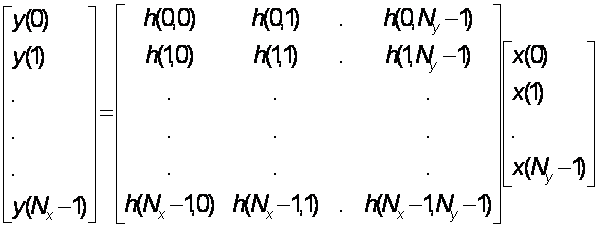References:

1. Jandel M., Morháč M., Kliman J., Krupa L., Matouek V., Hamilton J. H., Ramaya A. V.: Decomposition of continuum gamma-ray spectra using synthetized response matrix. NIM A 516 (2004), 172-183.

Example of unfolding:

Example 13 - script Unfolding.c:Fig. 20 Response matrix composed of neutron spectra of pure chemical elements.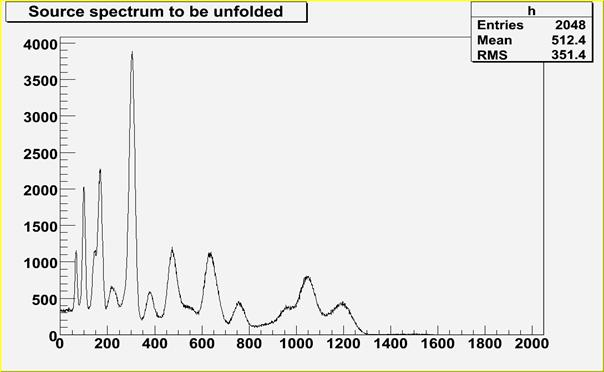Fig. 21 Source neutron spectrum to be decomposed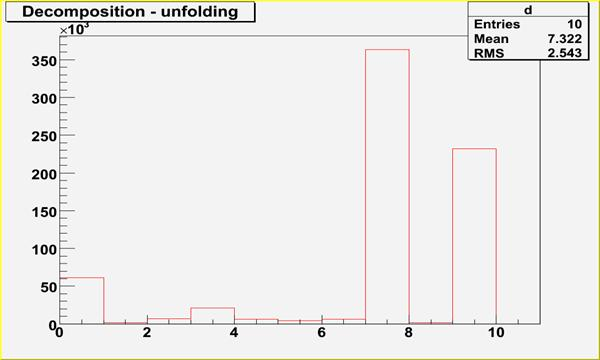Fig. 22 Spectrum after decomposition, contains 10 coefficients, which correspond to contents of chemical components (dominant 8-th and 10-th components, i.e. O, Si)

Script:

```   // Example to illustrate unfolding function (class TSpectrum).
// To execute this example, do
// root > .x Unfolding.C
#include
void Unfolding() {
Int_t i, j;
Int_t nbinsx = 2048;
Int_t nbinsy = 10;
Double_t xmin  = 0;
Double_t xmax  = (Double_t)nbinsx;
Double_t ymin  = 0;
Double_t ymax  = (Double_t)nbinsy;
Float_t * source = new float[nbinsx];
Float_t ** response = new float *[nbinsy];
for (i=0;iGet("decon_unf_in;1");
TFile *fr = new TFile("spectra\\TSpectrum.root");
decon_unf_resp = (TH2F*) fr->Get("decon_unf_resp;1");
for (i = 0; i < nbinsx; i++) source[i] = h->GetBinContent(i + 1);
for (i = 0; i < nbinsy; i++){
for (j = 0; j< nbinsx; j++){
response[i][j] = decon_unf_resp->GetBinContent(i + 1, j + 1);
}
}
TCanvas *Decon1 = gROOT->GetListOfCanvases()->FindObject("Decon1");
if (!Decon1) Decon1 = new TCanvas("Decon1","Decon1",10,10,1000,700);
h->Draw("L");
TSpectrum *s = new TSpectrum();
s->Unfolding(source,response,nbinsx,nbinsy,1000,1,1);
for (i = 0; i < nbinsy; i++) d->SetBinContent(i + 1,source[i]);
d->SetLineColor(kRed);
d->SetAxisRange(0,nbinsy);
d->Draw("");
}
```
```
```
Int_t SearchHighRes(float* source, float* destVector, Int_t ssize, float sigma, Double_t threshold, bool backgroundRemove, Int_t deconIterations, bool markov, Int_t averWindow)
One-dimensional high-resolution peak search function

This function searches for peaks in source spectrum. It is based on deconvolution method. First the background is removed (if desired), then Markov smoothed spectrum is calculated (if desired), then the response function is generated according to given sigma and deconvolution is carried out. The order of peaks is arranged according to their heights in the spectrum after background elimination. The highest peak is the first in the list. On success it returns number of found peaks.

Function parameters:

• source: pointer to the vector of source spectrum.
• destVector: pointer to the vector of resulting deconvolved spectrum.
• ssize: length of source spectrum.
• sigma: sigma of searched peaks, for details we refer to manual.
• threshold: threshold value in % for selected peaks, peaks with amplitude less than threshold*highest_peak/100 are ignored, see manual.
• backgroundRemove: logical variable, set if the removal of background before deconvolution is desired.
• deconIterations-number of iterations in deconvolution operation.
• markov: logical variable, if it is true, first the source spectrum is replaced by new spectrum calculated using Markov chains method.
• averWindow: averanging window of searched peaks, for details we refer to manual (applies only for Markov method).

Peaks searching:

The goal of this function is to identify automatically the peaks in spectrum with the presence of the continuous background and statistical fluctuations - noise.

The common problems connected with correct peak identification are:

• non-sensitivity to noise, i.e., only statistically relevant peaks should be identified.
• non-sensitivity of the algorithm to continuous background.
• ability to identify peaks close to the edges of the spectrum region. Usually peak finders fail to detect them.
• resolution, decomposition of doublets and multiplets. The algorithm should be able to recognize close positioned peaks.
• ability to identify peaks with different sigma.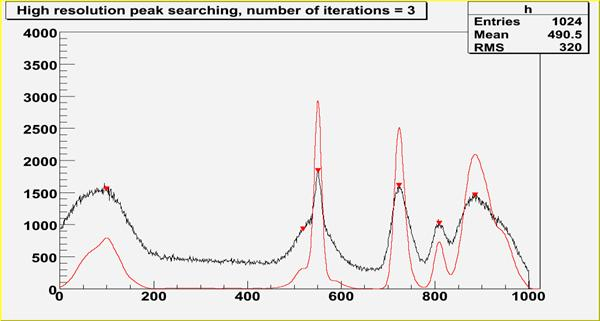Fig. 27 An example of one-dimensional synthetic spectrum with found peaks denoted by markers.

References:

1. M.A. Mariscotti: A method for identification of peaks in the presence of background and its application to spectrum analysis. NIM 50 (1967), 309-320.
2. M. Morháč, J. Kliman, V. Matouek, M. Veselský, I. Turzo.:Identification of peaks in multidimensional coincidence gamma-ray spectra. NIM, A443 (2000) 108-125.
3. Z.K. Silagadze, A new algorithm for automatic photopeak searches. NIM A 376 (1996), 451.

Examples of peak searching method:

The SearchHighRes function provides users with the possibility to vary the input parameters and with the access to the output deconvolved data in the destination spectrum. Based on the output data one can tune the parameters.

Example 15 - script SearchHR1.c:Fig. 28 One-dimensional spectrum with found peaks denoted by markers, 3 iterations steps in the deconvolution.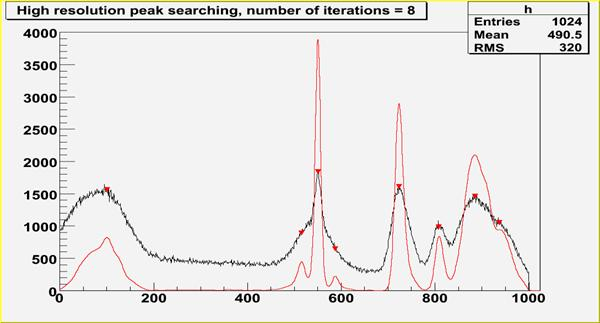Fig. 29 One-dimensional spectrum with found peaks denoted by markers, 8 iterations steps in the deconvolution.

Script:

```   // Example to illustrate high resolution peak searching function (class TSpectrum).
// To execute this example, do
// root > .x SearchHR1.C
#include
void SearchHR1() {
Float_t fPositionX;
Float_t fPositionY;
Int_t fNPeaks = 0;
Int_t i,nfound,bin;
Double_t nbins = 1024,a;
Double_t xmin  = 0;
Double_t xmax  = (Double_t)nbins;
Float_t * source = new float[nbins];
Float_t * dest = new float[nbins];
TH1F *h = new TH1F("h","High resolution peak searching, number of iterations = 3",nbins,xmin,xmax);
TH1F *d = new TH1F("d","",nbins,xmin,xmax);
TFile *f = new TFile("spectra\\TSpectrum.root");
h=(TH1F*) f->Get("search2;1");
for (i = 0; i < nbins; i++) source[i]=h->GetBinContent(i + 1);
TCanvas *Search = gROOT->GetListOfCanvases()->FindObject("Search");
if (!Search) Search = new TCanvas("Search","Search",10,10,1000,700);
h->SetMaximum(4000);
h->Draw("L");
TSpectrum *s = new TSpectrum();
nfound = s->SearchHighRes(source, dest, nbins, 8, 2, kTRUE, 3, kTRUE, 3);
Float_t *xpeaks = s->GetPositionX();
for (i = 0; i < nfound; i++) {
a=xpeaks[i];
bin = 1 + Int_t(a + 0.5);
fPositionX[i] = h->GetBinCenter(bin);
fPositionY[i] = h->GetBinContent(bin);
}
TPolyMarker * pm = (TPolyMarker*)h->GetListOfFunctions()->FindObject("TPolyMarker");
if (pm) {
h->GetListOfFunctions()->Remove(pm);
delete pm;
}
pm = new TPolyMarker(nfound, fPositionX, fPositionY);
pm->SetMarkerStyle(23);
pm->SetMarkerColor(kRed);
pm->SetMarkerSize(1.3);
for (i = 0; i < nbins; i++) d->SetBinContent(i + 1,dest[i]);
d->SetLineColor(kRed);
d->Draw("SAME");
printf("Found %d candidate peaks\n",nfound);
for(i=0;i

Example 16 - script SearchHR3.c:

Peak #  Position  Sigma
1       118       26
2       162       41
3       310       4
4       330       8
5       482       22
6       491       26
7       740       21
8       852       15
9       954       12
10      989       13

Table 4 Positions and sigma of peaks in the following examples.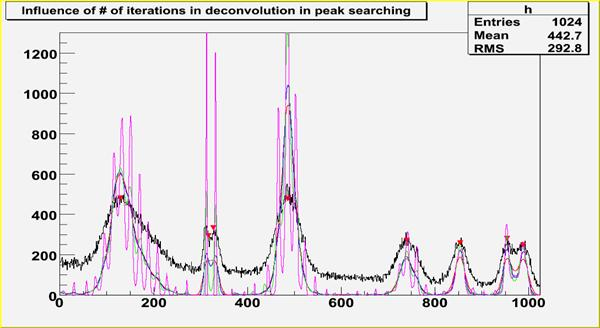Fig. 30 Influence of number of iterations (3-red, 10-blue, 100- green,
1000-magenta), sigma=8, smoothing width=3.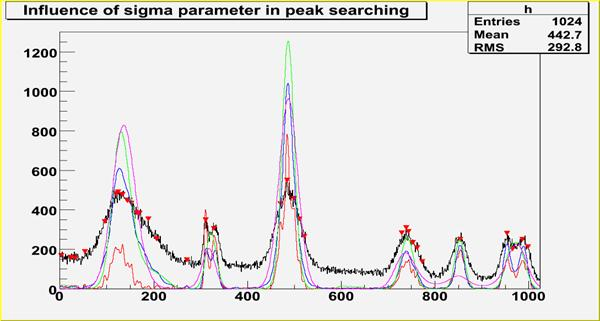Fig. 31 Influence of sigma (3-red, 8-blue, 20- green, 43-magenta),
num. iter.=10, sm. width=3.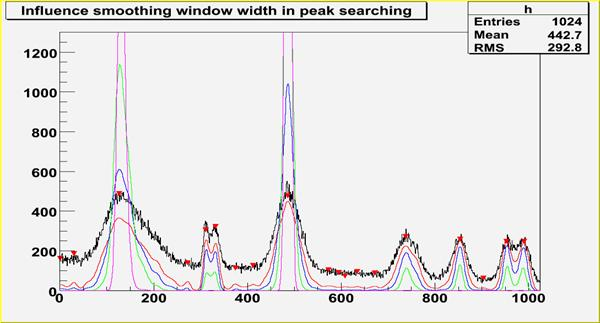Fig. 32 Influence smoothing width (0-red, 3-blue, 7- green, 20-magenta), num.
iter.=10, sigma=8.

Script:
// Example to illustrate the influence of number of iterations in deconvolution in high resolution peak searching function (class TSpectrum).
// To execute this example, do
// root > .x SearchHR3.C
#include
void SearchHR3() {
Float_t fPositionX;
Float_t fPositionY;
Int_t fNPeaks = 0;
Int_t i,nfound,bin;
Double_t nbins = 1024,a;
Double_t xmin  = 0;
Double_t xmax  = (Double_t)nbins;
Float_t * source = new float[nbins];
Float_t * dest = new float[nbins];
TH1F *h = new TH1F("h","Influence of # of iterations in deconvolution in peak searching",nbins,xmin,xmax);
TH1F *d1 = new TH1F("d1","",nbins,xmin,xmax);
TH1F *d2 = new TH1F("d2","",nbins,xmin,xmax);
TH1F *d3 = new TH1F("d3","",nbins,xmin,xmax);
TH1F *d4 = new TH1F("d4","",nbins,xmin,xmax);
TFile *f = new TFile("spectra\\TSpectrum.root");
h=(TH1F*) f->Get("search3;1");
for (i = 0; i < nbins; i++) source[i]=h->GetBinContent(i + 1);
TCanvas *Search = gROOT->GetListOfCanvases()->FindObject("Search");
if (!Search) Search = new TCanvas("Search","Search",10,10,1000,700);
h->SetMaximum(1300);
h->Draw("L");
TSpectrum *s = new TSpectrum();
nfound = s->SearchHighRes(source, dest, nbins, 8, 2, kTRUE, 3, kTRUE, 3);
Float_t *xpeaks = s->GetPositionX();
for (i = 0; i < nfound; i++) {
a=xpeaks[i];
bin = 1 + Int_t(a + 0.5);
fPositionX[i] = h->GetBinCenter(bin);
fPositionY[i] = h->GetBinContent(bin);
}
TPolyMarker * pm = (TPolyMarker*)h->GetListOfFunctions()->FindObject("TPolyMarker");
if (pm) {
h->GetListOfFunctions()->Remove(pm);
delete pm;
}
pm = new TPolyMarker(nfound, fPositionX, fPositionY);
pm->SetMarkerStyle(23);
pm->SetMarkerColor(kRed);
pm->SetMarkerSize(1.3);
for (i = 0; i < nbins; i++) d1->SetBinContent(i + 1,dest[i]);
h->Draw("");
d1->SetLineColor(kRed);
d1->Draw("SAME");
for (i = 0; i < nbins; i++) source[i]=h->GetBinContent(i + 1);
s->SearchHighRes(source, dest, nbins, 8, 2, kTRUE, 10, kTRUE, 3);
for (i = 0; i < nbins; i++) d2->SetBinContent(i + 1,dest[i]);
d2->SetLineColor(kBlue);
d2->Draw("SAME");
for (i = 0; i < nbins; i++) source[i]=h->GetBinContent(i + 1);
s->SearchHighRes(source, dest, nbins, 8, 2, kTRUE, 100, kTRUE, 3);
for (i = 0; i < nbins; i++) d3->SetBinContent(i + 1,dest[i]);
d3->SetLineColor(kGreen);
d3->Draw("SAME");
for (i = 0; i < nbins; i++) source[i]=h->GetBinContent(i + 1);
s->SearchHighRes(source, dest, nbins, 8, 2, kTRUE, 1000, kTRUE, 3);
for (i = 0; i < nbins; i++) d4->SetBinContent(i + 1,dest[i]);
d4->SetLineColor(kMagenta);
d4->Draw("SAME");
printf("Found %d candidate peaks\n",nfound);
}

```
Int_t Search1HighRes(float* source, float* destVector, Int_t ssize, float sigma, Double_t threshold, bool backgroundRemove, Int_t deconIterations, bool markov, Int_t averWindow)
Old name of SearcHighRes introduced for back compatibility.
This function will be removed after the June 2006 release

```
```
Int_t StaticSearch(const TH1* hist, Double_t sigma = 2, Option_t* option = "goff", Double_t threshold = 0.05)
Static function, interface to TSpectrum::Search.

```
```
TH1 * StaticBackground(const TH1* hist, Int_t niter = 20, Option_t* option = "")
Static function, interface to TSpectrum::Background.

```
```
TSpectrum(const TSpectrum& )
TSpectrum& operator=(const TSpectrum& )
TH1 * GetHistogram() const
`{return fHistogram;}`
Int_t GetNPeaks() const
`{return fNPeaks;}`
Float_t * GetPositionX() const
`{return fPositionX;}`
Float_t * GetPositionY() const
`{return fPositionY;}`